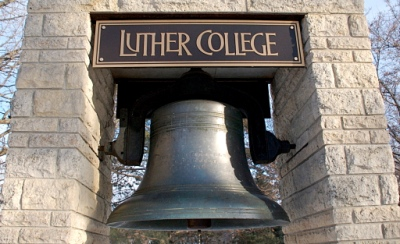# 8.14. Exercises¶

1. Add a print statement to Newton’s sqrt function that prints out better each time it is calculated. Call your modified function with 25 as an argument and record the results.

1. Write a function print_triangular_numbers(n) that prints out the first n triangular numbers. A call to print_triangular_numbers(5) would produce the following output:

1       1
2       3
3       6
4       10
5       15


(hint: use a web search to find out what a triangular number is.)

1. Write a function, is_prime, that takes a single integer argument and returns True when the argument is a prime number and False otherwise.

1. Modify the walking turtle program so that rather than a 90 degree left or right turn the angle of the turn is determined randomly at each step.

1. Modify the turtle walk program so that you have two turtles each with a random starting location. Keep the turtles moving until one of them leaves the screen.

1. Modify the previous turtle walk program so that the turtle turns around when it hits the wall or when one turtle collides with another turtle (when the positions of the two turtles are closer than some small number).

1. Write a function to remove all the red from an image.#### For this and the following exercises, use the luther.jpg photo.

1. Write a function to convert the image to grayscale.

1. Write a function to convert an image to black and white.

1. Sepia Tone images are those brownish colored images that may remind you of times past. The formula for creating a sepia tone is as follows:

newR = (R × 0.393 + G × 0.769 + B × 0.189)
newG = (R × 0.349 + G × 0.686 + B × 0.168)
newB = (R × 0.272 + G × 0.534 + B × 0.131)


Write a function to convert an image to sepia tone. Hint: Remember that rgb values must be integers between 0 and 255.

1. Write a function to uniformly enlarge an image by a factor of 2 (double the size).